### 一、题目描述

输入：x = 2.00000, n = 10



输入：x = 2.10000, n = 3



输入：x = 2.00000, n = -2



• -100.0 < x < 100.0
• -231 <= n <= 231-1
• -104 <= xn <= 104

### 二、题目解析

• 模拟：模拟题目的运行。
• 规律：尝试总结出题目的一般规律和特点。
• 匹配：找到符合这些特点的数据结构与算法。
• 边界：考虑特殊情况。

#### 1、模拟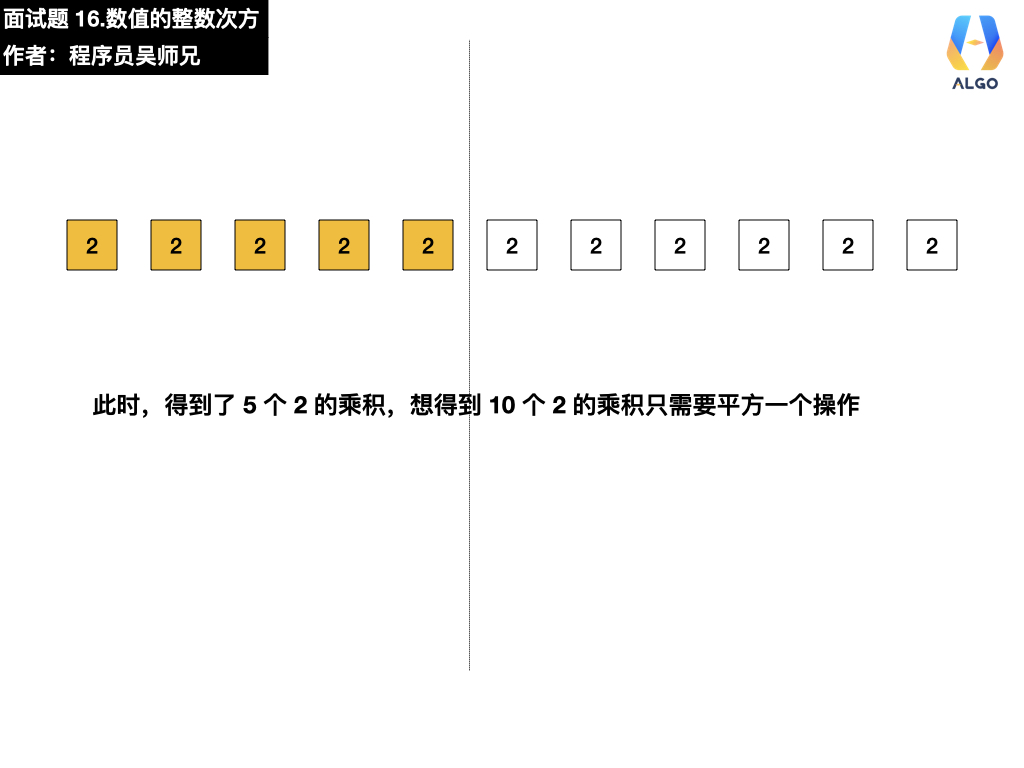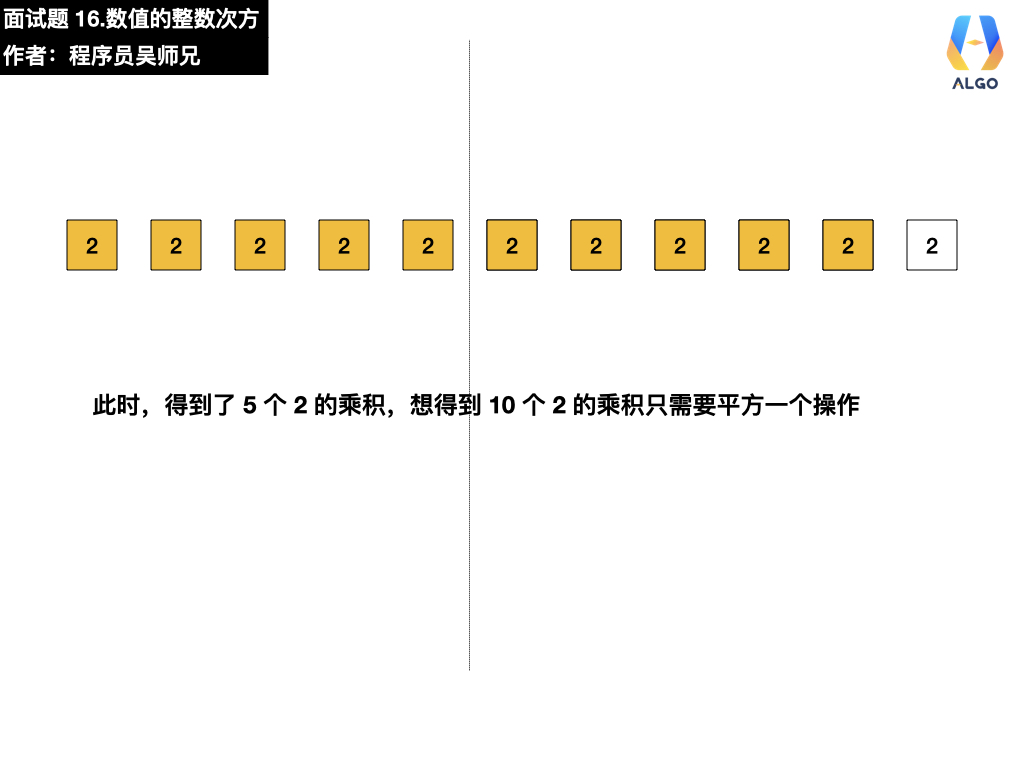### 三、动画描述

• 普通用户特权：5积分
• 会员用户特权：免费
• 算法训练营永久会员用户特权：免费推荐

### 四、图片描述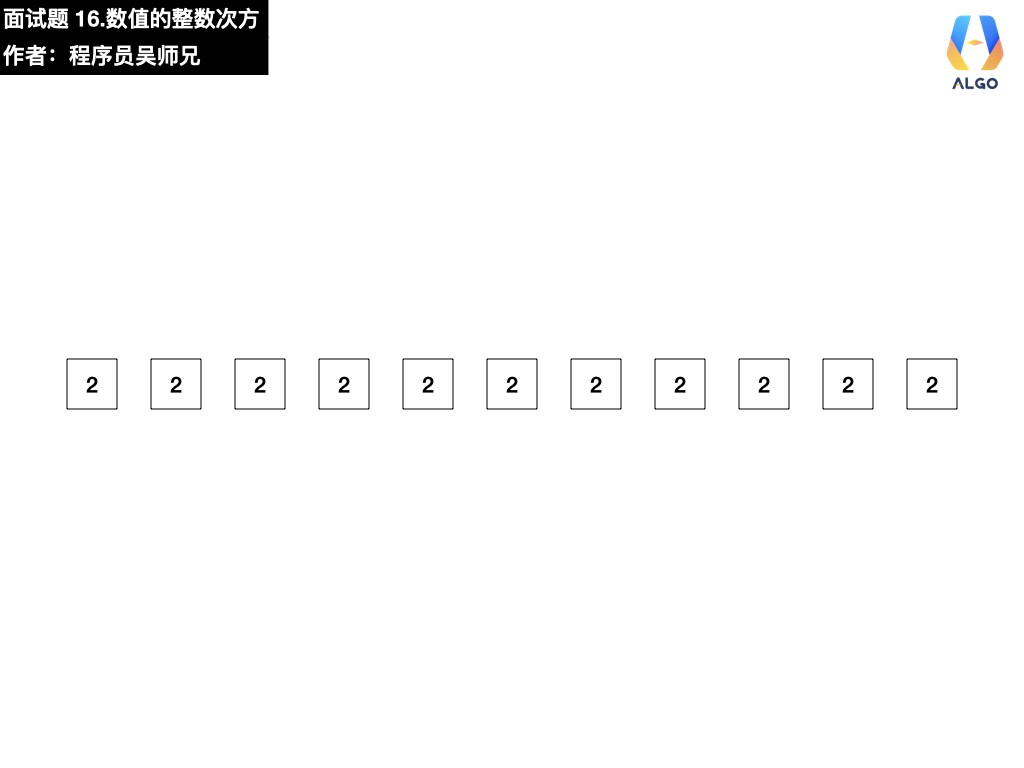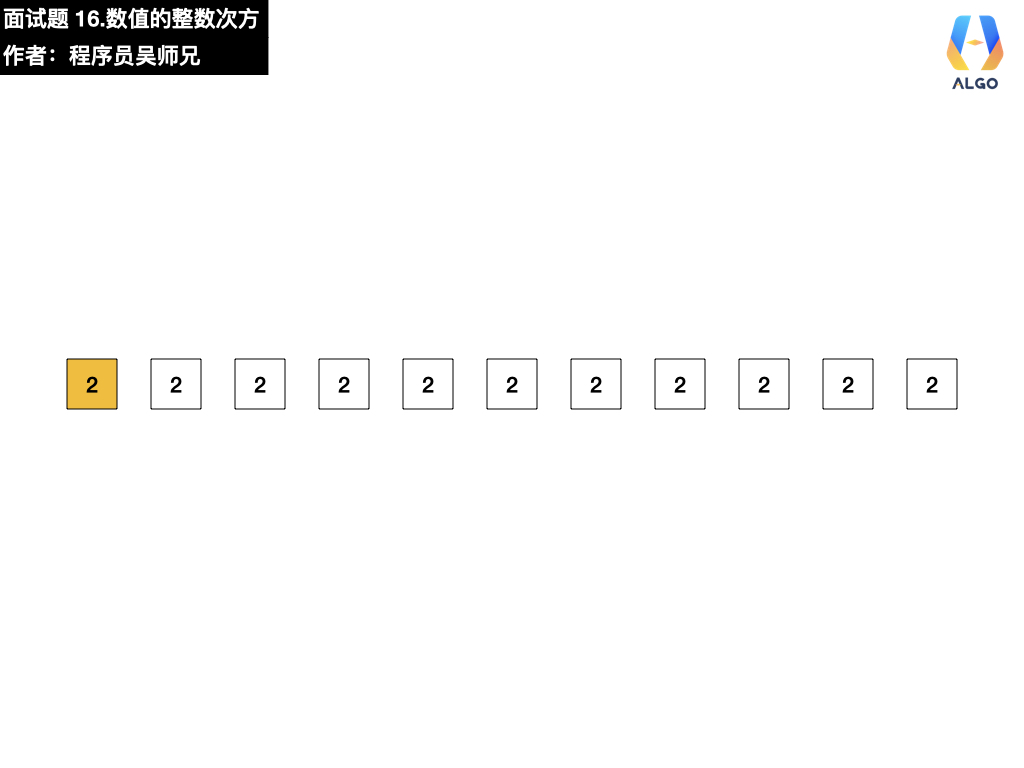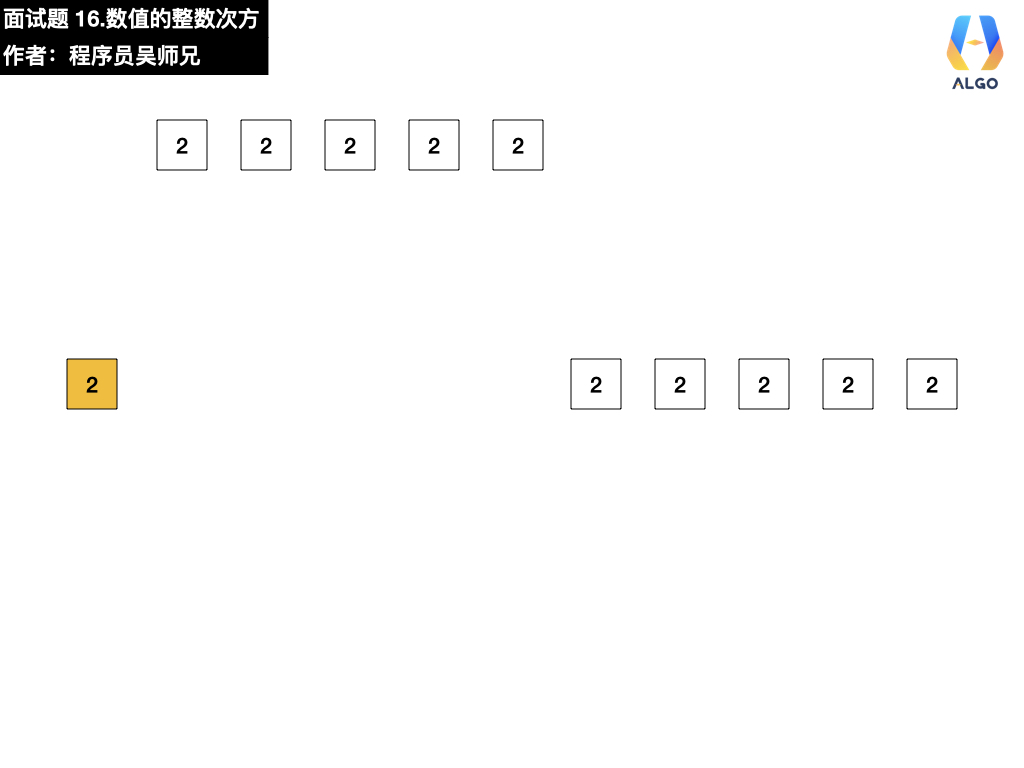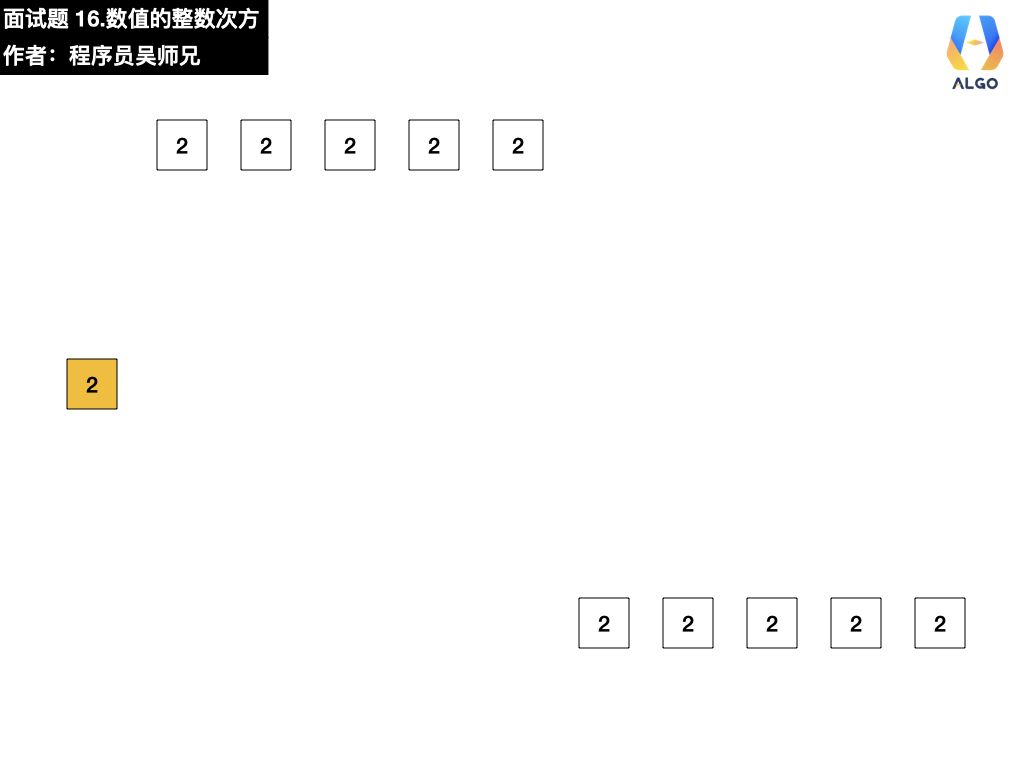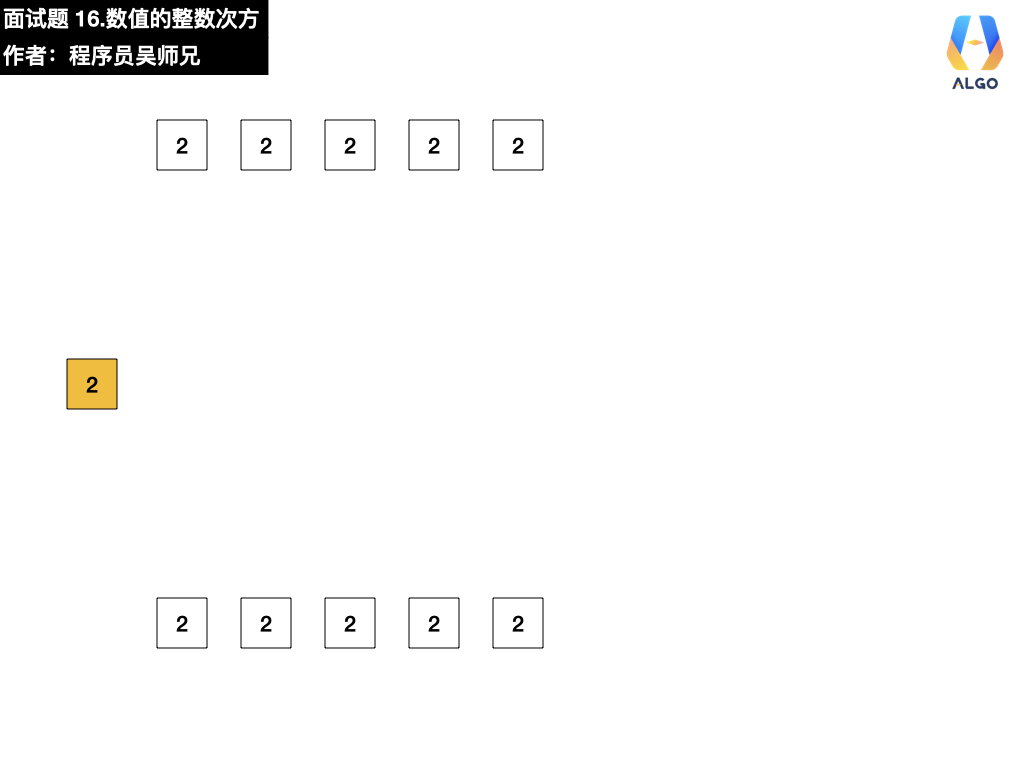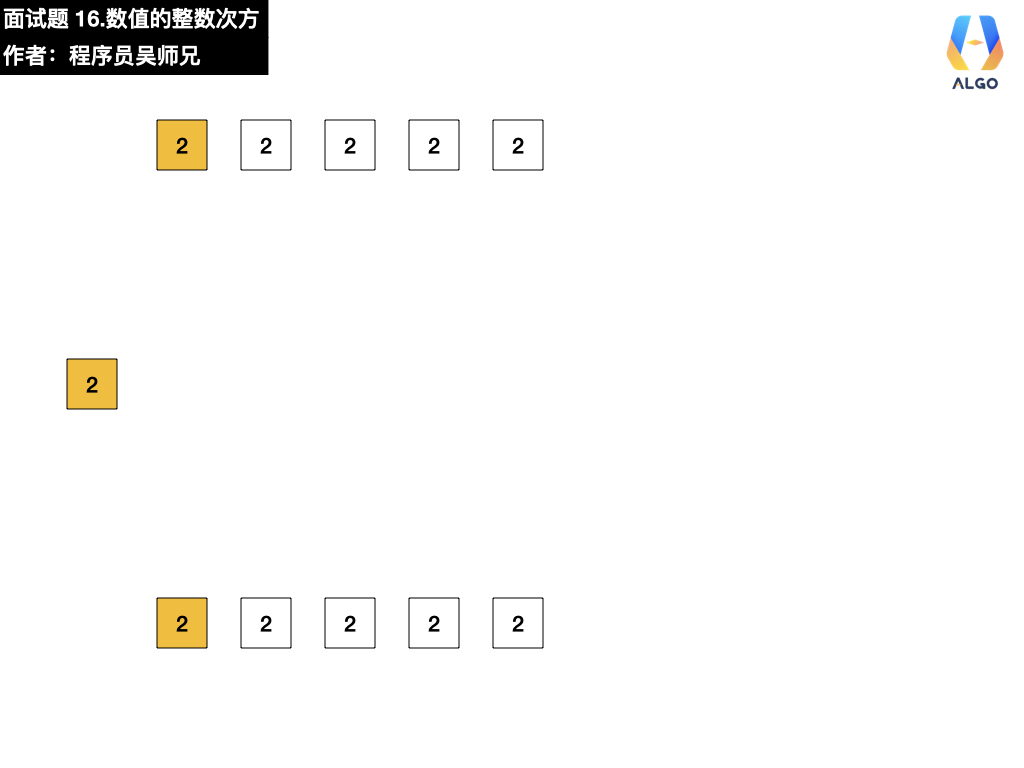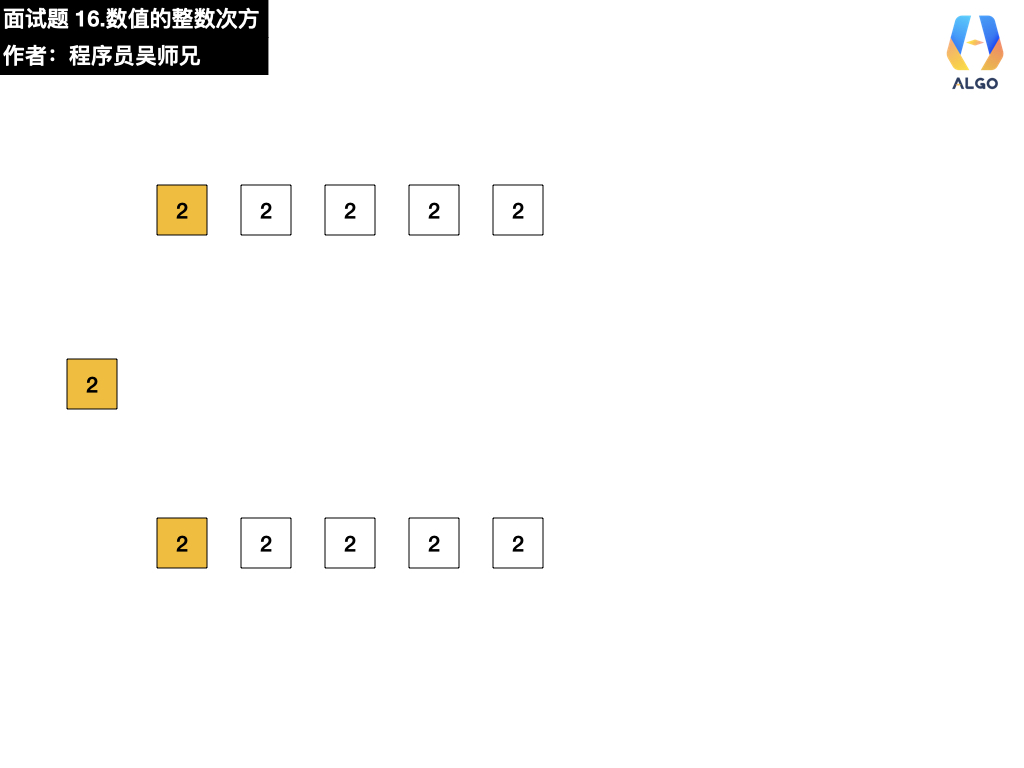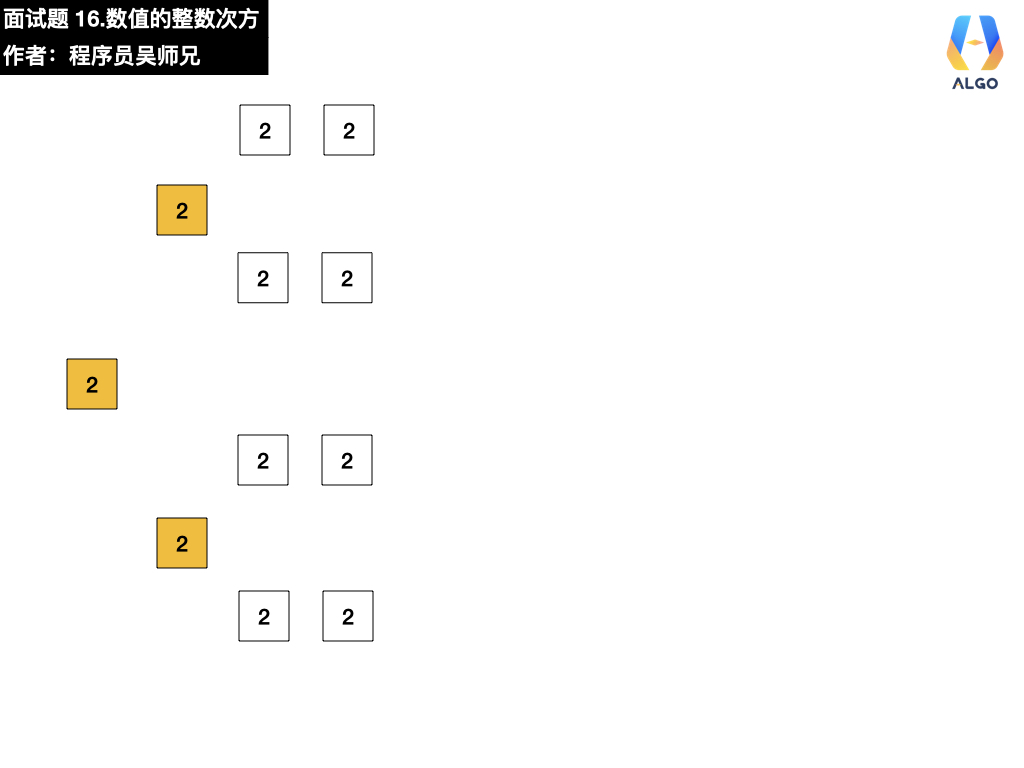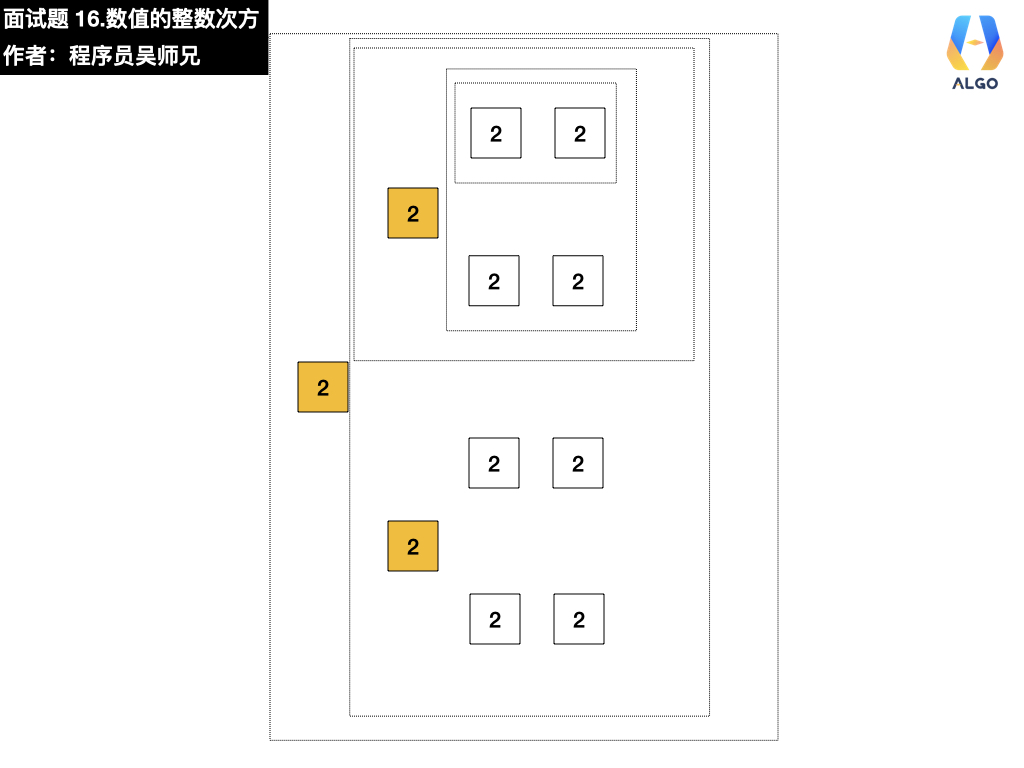### 五、参考代码

// 登录 AlgoMooc 官网获取更多算法图解
// https://www.algomooc.com
class Solution {
public double myPow(double x, int n) {
//当 n = 0 时，返回 1
if(n == 0){
return 1;
}else if(n < 0){
// 当 n < 0 时，返回 1/ x^-n
return 1 / (x * myPow(x, - n - 1));
}else if(n % 2 == 1){
//为避免越界，提取 x
return x * myPow(x, n - 1);
}else{
return myPow(x * x, n / 2);
}
}
}


• 递归